# Introduction.

The purpose of this tutorial is to illustrate the difference between streamlines, streak lines and path lines for a time dependent velocity field using the java application called vector field manipulator. In this application the user can type the equations of the vector field to be studied. Then he click the button draw, and the vector field is displayed. By mouse clicking in the display area he can input a point to the application. This point can be the initial point to trace a streamline passing through it, or the path line starting at it, or the streak line starting at it. The user can chose the options streamline, path line and streak line from the drop down menu box. Once the user makes his/her choice and click the draw button, the choice made became active.

When we study a fluid flow, we are usually interested in the fluid velocity at points in space. The collection of the velocity vectors over a grid in the fluid domain is a vector field (velocity field). When we talk about streamlines, streak lines and path lines of a fluid flow, we are talking about properties of the velocity field of the flow in question. Next, we give a more rigorous definition of the streamlines, path lines and streak lines.

# Streamlines

The streamlines are solutions of the equations: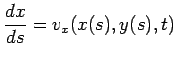and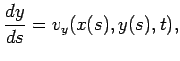with initial conditions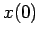and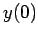(the point given by the user by mouse clicking in the display area), andis the parameterization of the vector field. We integrate the equations above for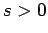and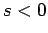to obtain the streamline that pass through the point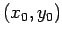. Notice that the streamlines are given at a given value of time (the value of time inputed in the box Input t). We take a picture of the vector field at the particular instant we want, and we trace the lines tangent to the vectors. That is exactly what we do in the application with this option. For time dependent vector fields, different values ofgive different vector fields, and therefore, different streamlines.

To visualize the difference between streamlines for a time dependent and a time independent vector field, we are going to do an exercise using the vector field manipulator java application. First, we consider the time independent vector field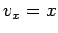and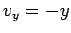Choose the streamline option from the drop down menu box, and click the draw button. Then click in the display are to get, let say three different streamlines. Next, check the box change streamlines in time, then change the value of time in the input t box and click the draw button. The streamlines change colors, but they are the same for any instant we input in the input t box. Input one more value for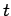in the input t box, and then click the draw button. Next, we consider a time dependent vector field, given by the equations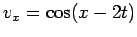and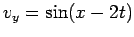Repeat the exercise above. In this case, the streamlines change with time since the vector field form change with time.

# Path lines

The path lines are solutions of the equations: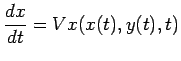and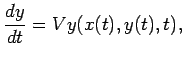with initial conditionsand(the point given by the user by mouse clicking in the display area). To obtain the path line that started at the point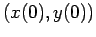, we integrate the equations above forward in time (integrate for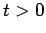). Notice the difference between the equations defining streamlines and path lines.

We are going to do a similar exercise as in the previous section. Consider again the time independent vector fieldandFirst, chose the option streamline from the drop down menu box, and click the draw button. Chose three points from the grid to get three streamlines. Remember the points you chose to trace streamlines. Next, chose the option path line from the drop down menu box, and click the draw button. The streamlines are gone, but try to remember their shape. Now, chose the same three points you used before to trace path lines. Notice that they are basically the streamlines. Actually the part of the streamlines that resulted from the forward integration in time of the vector field equations. Then, for a time independent vector field, the path lines coincide with part of the streamlines.

Next, we repeat the exercise, but now we use again the time dependent vector fieldandYou will see how different the path lines are from the streamlines. There is no resemblance with the path lines and streamlines.

# Streak lines

According to the lecture note, it is the instantaneous locus of all particles that pass a given point. Given a point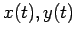, the streak line starting at this point is the set of points given by the equation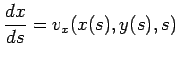and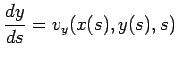with initial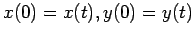for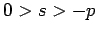with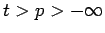. If we just want some points of the streak line, we can with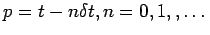.

Next, we do an exercise to compute a streak line for a time independent vector field. We will see that the streak line starting at a chosen point coincides with the path line starting at this point and with the streamline passing through this point for the case of a time independent vector field. We again consider the vector fieldandFirst, chose the option streamline from the drop down menu box, and click the draw button. Chose one point from the grid to get three streamlines. Remember the point you chose to trace the streamlines. Second, chose the option path line from the drop down menu box, and click the draw button. The streamline is gone, but try to remember its shape. Now, chose the same point you used before,and trace the path line. As expected, path line and streamline are the same. Third, chose the option streak line from the drop down menu box, and click the draw button. Use the same point as you used for the path and streamline case. You will see that the streak line for the time independent vector field coincides with the path line starting at the chosen point. In summary, for time independent vector field, the path lines coincide with the streak lines, and they coincide with part of the streamlines.

Next, we consider the time dependent vector fieldandRepeat the exercise described in the paragraph above. You will see that for time dependent vector field , the streamlines, path lines and streak lines are different.

Karl P Burr 2003-08-23